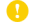Online ISSN : 1884-1082
Print ISSN : 0021-5236
ISSN-L : 0021-5236
Jastrow錯視に関する実験

ジャーナル フリー

1960 年 30 巻 5 号 p. 350-356[Correction] The Japanese Journal of Psychology Vol.31 No.2 (1960) pp.151a-151a[Correction] The Japanese Journal of Psychology Vol.30 No.6 (1960) pp.428-428

The experiments were designed to test the illusory effects of the various stimulus factors in Jastrow figure.
Variables were as follows: (1) Radius of inside and outside circular arcs, ri, ro. (2) Opening angle of sector, 2θ. (3) Angle of cut ends of sector, θ′. (Fig. 2). (4) Curvilinearity of circular arcs. (5) Distance between two sectors, d. (6) Spatial conditions of sectors placed in vertical or horizontal directions, v, v′, h, h′ (Fig. 3).
Results obtained were as follows:
A. Generally: The size (area) of the outside sector was always relatively underestimated. But, in the absolute quantity, this was mainly due to the expansion of the size of the inside sector. The maximum values of relative underestimation were about 10%.
B. Factors related to form: (1) The effect of the factor of the width between the concentric circular arcs, or the length of the cut ends, presented the mountain-shaped curve, and the maximum point appeared in ri/ro=3/5 (variations of condition; ri/ro=0/5-5/5). (2) The effect of the factor of the opening angle presented the mountain-shaped curve with a sharp summit, and the maximum point appeared in 2θ=40° (variations; 2θ=20°-180°). (3) The maximum effects of the factors of the angle or direction of the cut ends appeared in θ′=0° when 2θ=40°, 80°, 120°, and, in θ′=-50° when 2θ=180° (variations; θ′=0° -±90°).
Here, in these angular factors, involving the opening angle, two kinds of factors are assumed; one is the general angle (g-factor) and the other the specific (s-factor). The maximum effects of g-factor are in 2θ=20°-40°, and those of s-factor are when the prolongation of the cut ends passes the common center of the concentric circles, and the former effects are stronger than the latter. The effects of the factors of the cut ends could be accounted by the manner of the composition of these two (g, s) factors, in some cases the maximum points coinciding or overlapping, and in some cases shifting (Fig. 5). (4) The existence of the circular arcs also is essential to Jastrow illusion because the effect of the curvilinearity of the sectors is about twice, in maximum value, that of the linearity of the trapezoids.
C. Factors related to spatial arrangement: (5) The illusory effect of the vertical (v) condition was superior to the other conditions. (6) The amount of the relative under estimation of the outside sector decreased monotonously with the increase of the distance between the two sectors (variations; d=1-19cm).
After all, the specific effects in Jastrow illusion consist of direct regulating factors such as the direction and length of the cut ends and also such as the curvilinearity of the circular arcs. However, the effects of other indirect factors such as the vertical and horizontal conditions, and the distance between sectors cannot be neglected either.著者関連情報